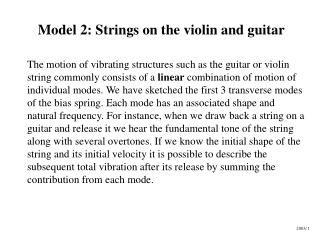DownloadDownload PresentationModel 2: Strings on the violin and guitar

# Model 2: Strings on the violin and guitar

Télécharger la présentation## Model 2: Strings on the violin and guitar

- - - - - - - - - - - - - - - - - - - - - - - - - - - E N D - - - - - - - - - - - - - - - - - - - - - - - - - - -
##### Presentation Transcript

1. Model 2: Strings on the violin and guitar The motion of vibrating structures such as the guitar or violin string commonly consists of a linear combination of motion of individual modes. We have sketched the first 3 transverse modes of the bias spring. Each mode has an associated shape and natural frequency. For instance, when we draw back a string on a guitar and release it we hear the fundamental tone of the string along with several overtones. If we know the initial shape of the string and its initial velocity it is possible to describe the subsequent total vibration after its release by summing the contribution from each mode. 2003/ 1

2. Step 1: Model the problem and find an equation A musical string can be modelled as an elastic string. We will use the same equation: (5) The 1 dimensional wave equation

3. Step 2: Derive a general solution This has also already been done. We use equation 8: (8)

4. L Step 3: Fit the solution to the boundary conditions The boundary conditions are the same as before for the spring: At x=0 u(0,t) = 0 Thus B= 0 At x=L u(L,t) = 0 So sin(L) = 0 L = nπwhere n= 1,2,3,….

5. u3(x,t) u2(x,t) u1(x,t) As we found before---- (9) n= 1,2,3,…. and the rest.

6. Equation 9 describes the motion in each mode: (9) n= 1,2,3,…. The resultant motion on the string is the sum of modes described by eqn. 9: (10)

7. Step 4: Fit the solution to the initial conditionsWe will model a string that is plucked at the middle. At t=0 it will look like this: L/2 X=0 X=L

8. 0 1 Appropriate values for anand bn are determined by the initial conditions: the displacement and velocity at t=0. At t=0 u(x,0) only contains terms multiplied by bn: (11)

9. In a similar way we get terms with an only by writing the derivative of u at t=0: (10) Differentiate wrt t t=0 (12)

10. Summary:We match the initial conditions (displacement and velocity) to the solution at t=0 to evaluate the anand bn constants. (11) Initial displacement Initial velocity (12)

11. We have a problem! There are an infinite number of an‘sand bn ‘s. How do we evaluate all of them? We can use a property of the sine function: if n=m if n ≠ m(13)

12. The trick is to multiply both sides of equations 11 and 12 by sin (mπx) and then integrate between the limits 0 and L. All terms are equal to zero except those where n =m. We can do this to eqn. 11: (14) Rearrange to find the value of the m’th term: (15)

13. We can also obtain the following expression for the an terms using equation 12: (12) (16)

14. Back to our example: We assume that the guitar string has been pulled back 0.5 L at its middle and then released at t=0. L/2 0.5L X=0 X=L Initial conditions: for x=0 to L/2 (17) for x=L/2 to L (18) for x=0 to L (19)

15. Evaluate all bn ‘s. Use eqn. 15 to evaluate m’th term

16. Evaluate all an ‘s. Use eqn. 16 to evaluatethe m’th term 0 (16) The velocity is zero at t=0 and we can see from eqn. 16 that all an = 0 too.

17. The final solution: Plucked string at t=0 (left) and the first 3 non-zero modes that sum to produce the total motion.

18. The bow and string String capture Release Capture

19. String motion: travelling “kink” moves at speed c=(T/) String capture Release

20. This causes the bridge to rock The rocking action forces the body to vibrate and sound is radiated.

21. Violin tuning question hint: Consider the E and A strings: Fundamental of A is tuned to 440 Hz E string fundamental is around but not exactly 660Hz. Beat frequency between fundamentals would be approximately 660-440= 220 Hz. Yet we hear beating of the order of a 1 to 10 Hz as we adjust the E string. This beat frequency reduces to zero as we improve the tuning.